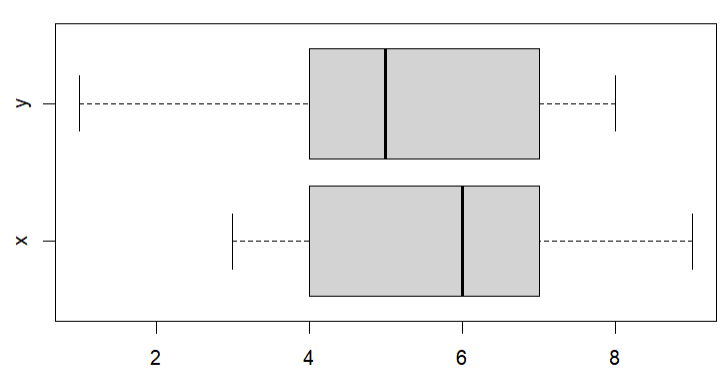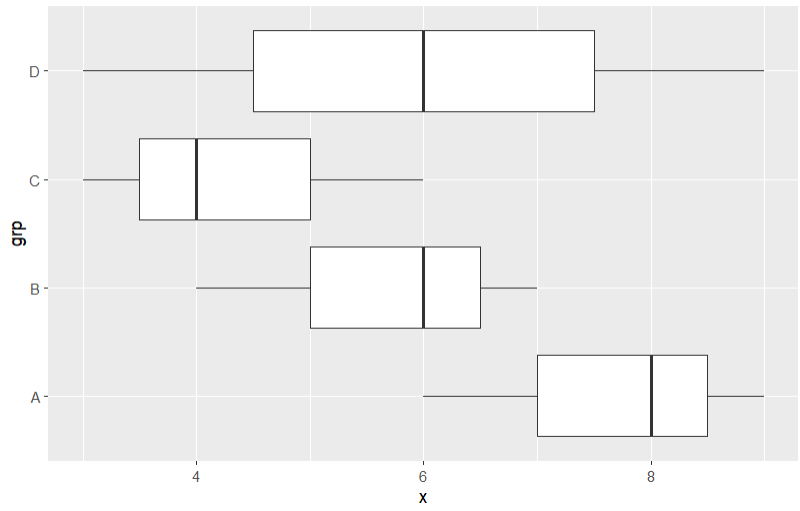# How to Create Horizontal Boxplots in R?

• Last Updated : 19 Dec, 2021

In this article, we will discuss how to create horizontal boxplots in the R programming language.

## Method 1: Create Horizontal boxplot in base R

In this method to create the horizontal bar plot, the user simply needs to call the boxplot() function which is a base function of the R language, then the user needs to call the horizontal argument of this function and initialize it with true value to get the box plot in a horizontal way.

boxplot() function: This function is used to produce box-and-whisker plot(s) of the given values.

Syntax: boxplot(formula, data = NULL, …, horizontal = TRUE)

Parameters:

• formula: a formula, such as y ~ grp, where y is a numeric vector of data values to be split into groups according to the grouping variable grp.
• data: a data.frame (or list) from which the variables in the formula should be taken.
• horizontal: logical indicating if the boxplots should be horizontal.
• … : Other parameters

Example: In this example, we are taking a data frame with 2 variables to create the horizontal boxplot of the given variable using the boxplot with a horizontal argument with it in the R programming language.

## R

 `# Create DataFrame ` `gfg<-``data.frame``(x=``c``(6,8,9,6,4,7,6,3,4), ` `                ``y=``c``(4,6,8,7,8,4,5,1,3)) ` ` `  `# Create Horizontal Boxplot ` `boxplot``(gfg,horizontal=``TRUE``)`

Output:## Method 2: Create a Horizontal boxplot using coord_flip()

In this method to create the horizontal boxplot in ggplot2, the user needs to install and import the ggplot2 package in the working R console, here the ggplot2 package is responsible to plot the boxplot and provide other functionalities of the package. Then the user needs to simply call the geom_boxplot() function which will be simply plotting the boxplot in the vertical way of the given data and with it the user also needs to call the coord_flip() which will be flipping the axis and the final boxplot will be resulting in the horizontal boxplot in the R programming language.

Syntax to import and install ggplot2 package in R language:

```install.package("ggplot2")
library("ggplot2")```

coord_flip() function: This function is used to flip cartesian coordinates so that horizontal becomes vertical, and vertical, horizontal.

Syntax: coord_flip(…)

Parameters:

• …: Other arguments passed

Example: In this example, we will be using the geom_boxplot() function with the coord_flip() function from the ggplot2 package to plot the horizontal boxplot of the given data in the R programming language.

## R

 `# Import ggplot2 package ` `library``(ggplot2) ` ` `  `# Create Data ` `gfg<-``data.frame``(x=``c``(6,8,9,6,4,7,6,3,4,9,6,3), ` `                ``grp=``rep``(``c``(``'A'``,``'B'``,``'C'``,``'D'``), ` `                        ``each=3)) ` ` `  `# Create horizontal boxplot ` `ggplot``(gfg,``aes``(x=grp,y=x)) +``geom_boxplot``() + ``coord_flip``() `

Output:My Personal Notes arrow_drop_up
Recommended Articles
Page :